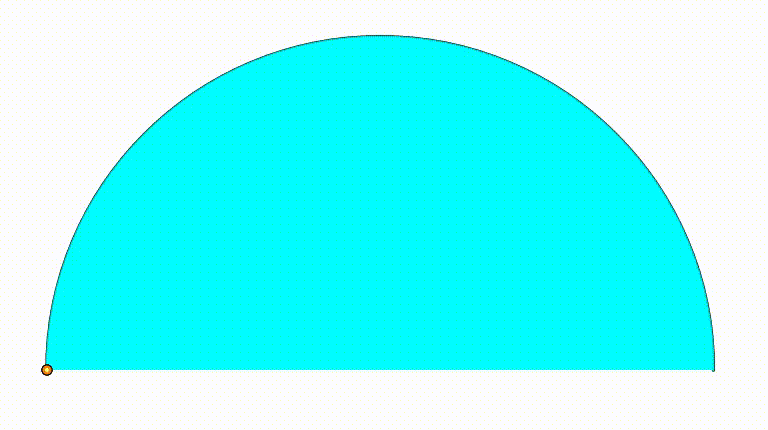# Dynamic Geometry: P105

Geometry Level 4The diagram shows a black semicircle with radius $1$. The cyan and green semicircle are growing and shrinking freely on the black semicirle's diameter. The purple circle is internally tangent to the black semicircle and tangent to both green and cyan semicircle. The red and orange circles are internally tangent to the black semicircle and tangent to the purple circle and to one of the bottom semicircles.Using the tangency points, we draw a blue and an yellow triangle. When the sum of the curvature of each circle (purple, red, orange) is equal to $\dfrac{211}{8}$, the ratio of the area of both triangles can be expressed as $\dfrac{p}{q}$, where $p$ and $q$ are coprime positive integers. Find $p+q$.

×